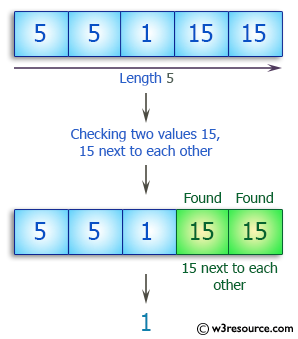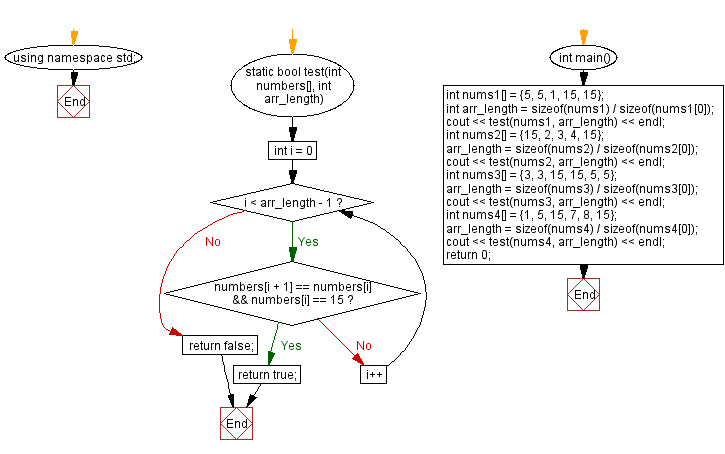﻿ C++ Exercises: Check a given array of integers and return true if there are two values 15, 15 next to each other - w3resource# C++ Exercises: Check a given array of integers and return true if there are two values 15, 15 next to each other

## C++ Basic Algorithm: Exercise-117 with Solution

Write a C++ program to check a given array (length will be atleast 2) of integers and return true if there are two values 15, 15 next to each other.

Sample Solution:

C++ Code :

``````#include <iostream>
using namespace std;

static bool test(int numbers[], int arr_length)
{
for (int i = 0; i < arr_length - 1; i++)
{
if (numbers[i + 1] == numbers[i] && numbers[i] == 15) return true;
}
return false;
}

int main()
{
int nums1[] = {5, 5, 1, 15, 15};
int arr_length = sizeof(nums1) / sizeof(nums1);
cout << test(nums1, arr_length) << endl;
int nums2[] = {15, 2, 3, 4, 15};
arr_length = sizeof(nums2) / sizeof(nums2);
cout << test(nums2, arr_length) << endl;
int nums3[] = {3, 3, 15, 15, 5, 5};
arr_length = sizeof(nums3) / sizeof(nums3);
cout << test(nums3, arr_length) << endl;
int nums4[] = {1, 5, 15, 7, 8, 15};
arr_length = sizeof(nums4) / sizeof(nums4);
cout << test(nums4, arr_length) << endl;
return 0;
}
``````

Sample Output:

```1
0
1
0
```

Pictorial Presentation:Flowchart:C++ Code Editor:

Contribute your code and comments through Disqus.

What is the difficulty level of this exercise?

﻿